# imaging::EquationInterface< fem_types > Class Template Reference [Equations]

Abstract base class of all PDEs to be solved using the finite element module. More...

#include <EquationInterface.hpp>

List of all members.

## Public Member Functions

std::size_t system_size () const
float_t stiffness_matrix (std::size_t k, std::size_t l, std::size_t i, std::size_t j, std::size_t integrator_node, const FemKernel< fem_types > &kernel) const
float_t force_vector (std::size_t k, std::size_t i, std::size_t integrator_node, const FemKernel< fem_types > &kernel) const
float_t stiffness_matrix_at_boundary (std::size_t k, std::size_t l, std::size_t i, std::size_t j, std::size_t integrator_node, const FemKernel< fem_types > &kernel) const
float_t force_vector_at_boundary (std::size_t k, std::size_t i, std::size_t integrator_node, const FemKernel< fem_types > &kernel) const
bool sanity_check_stiffnesss_matrix (const FemKernel< fem_types > &kernel, std::string &error_message) const
bool sanity_check_force_vector (const FemKernel< fem_types > &kernel, std::string &error_message) const

## Detailed Description

### template<class fem_types> class imaging::EquationInterface< fem_types >

Abstract base class of all PDEs to be solved using the finite element module.

To solve a (system of) PDEs with the imaging2 finite element module the user must design a class which implements the public members of this interface. It is also recommended to actually derive your class from EquationInterface. Then, an object of this class should be passed to Assembler::assemble(), Assembler::assemble_stiffness_matrix() or Assembler::assemble_force_vector() to compute the system of linear equation corresponding to the PDE.

The interface consists of four functions computing the coefficients in the stiffness matrix and the force vector respectively for a given element (determined by the current state of FemKernel). The function system_size() returns the number of equations in the system of PDEs.

For scalar PDEs it is advisable to make use of the interface SimpleEquationInterface. A simple equation can be transformed into a equation complying with EquationInterface by using SimpleEquationAdaptor.

To understand the parameters of the member function we give a (very technical) definition of the PDE problem this interface can model. Imagine that we are interested in the problem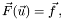where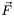depends on the vector-valued function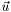and its (higher order) derivative. We will rewrite this equation as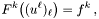where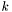and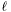are indices in the range of the number of equations of the system. Furthermore we assume a basis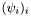of shape functions on the problem domain, where each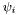corresponds to a node on the grid. We define the vector-valued shape functions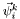as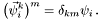Following the common finite element approach we we express the unknown functionin terms of the shape functions, i.e.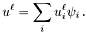We hit the above equation with each of the test functions and integrate the resulting equations: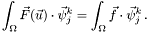Then we can write the above equation in the following form: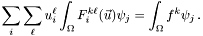Applying the Trace Theorem yields an extended version of this equation (to save symbols we re-use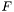and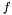; they are not the same functions as above):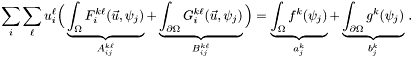The parts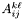,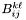,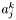and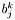then correspond to the four members we mentioned in the introduction. Note that the above version of the equation is not uniquely determined. It depends on how the user applies the Trace Theorem, i.e. which parts of the equation you chose to transport to the boundary.

If she also must incorporate boundary conditions she might choose not to evaluate the equation on the boundary at all. This is equivalent to hit the equation with test functions with compact support only. Then, the termsandvanish at first. Instead, consider boundary conditions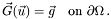and hit them with non-compactly supported test functions of the above form, i.e. test functionswhere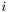refers to a boundary node. Performing the same procedure as above on this equation adds the termsandagain (this time as a result of the boundary equation and not of the Trace Theorem).

## Member Function Documentation

template<class fem_types>
 std::size_t imaging::EquationInterface< fem_types >::system_size ( ) const

Returns the number of equations in this system of PDEs. For scalar PDEs this is 1.

Reimplemented in imaging::SimpleEquationAdaptor< equation_t >.

template<class fem_types>
 float_t imaging::EquationInterface< fem_types >::stiffness_matrix ( std::size_t k, std::size_t l, std::size_t i, std::size_t j, std::size_t integrator_node, const FemKernel< fem_types > & kernel ) const

Computes the contribution of the integralin integrator_node on the current element of kernel. The node indices i and j are node indices on the element, not global indices.

As kernel is set to the current element, in the implementation of this function all relevant values of the shape functions and the element transform can be retrieved from kernel via the indices i, j and integrator_node. One can also obtain the FE grid from the kernel (FemKernel::grid()) and use its interpolation methods (passing the already correctly initialized kernel to them).

Reimplemented in imaging::SimpleEquationAdaptor< equation_t >.

template<class fem_types>
 float_t imaging::EquationInterface< fem_types >::force_vector ( std::size_t k, std::size_t i, std::size_t integrator_node, const FemKernel< fem_types > & kernel ) const

Computes the contribution of the integralin integrator_node on the current element of kernel. The node index i is the node index on the element, not a global index.

As kernel is set to the current element, in the implementation of this function all relevant values of the shape functions and the element transform can be retrieved from kernel via the indices i and integrator_node. One can also obtain the FE grid from the kernel (FemKernel::grid()) and use its interpolation methods (passing the already correctly initialized kernel to them).

Reimplemented in imaging::SimpleEquationAdaptor< equation_t >.

template<class fem_types>
 float_t imaging::EquationInterface< fem_types >::stiffness_matrix_at_boundary ( std::size_t k, std::size_t l, std::size_t i, std::size_t j, std::size_t integrator_node, const FemKernel< fem_types > & kernel ) const

Computes the contribution of the integralin integrator_node on the current boundary element of kernel. The node indices i and j are node indices on the element, not global indices.

As kernel is set to the current boundary element, in the implementation of this function all relevant values of the shape functions and the element transform can be retrieved from kernel via the indices i, j and integrator_node. One can also obtain the FE grid from the kernel (FemKernel::grid()) and use its interpolation methods (passing the already correctly initialized kernel to them).

Reimplemented in imaging::SimpleEquationAdaptor< equation_t >.

template<class fem_types>
 float_t imaging::EquationInterface< fem_types >::force_vector_at_boundary ( std::size_t k, std::size_t i, std::size_t integrator_node, const FemKernel< fem_types > & kernel ) const

Computes the contribution of the integralin integrator_node on the current element of kernel. The node index i is the node index on the element, not a global index.

As kernel is set to the current boundary element, in the implementation of this function all relevant values of the shape functions and the element transform can be retrieved from kernel via the indices i and integrator_node. One can also obtain the FE grid from the kernel (FemKernel::grid()) and use its interpolation methods (passing the already correctly initialized kernel to them).

Reimplemented in imaging::SimpleEquationAdaptor< equation_t >.

template<class fem_types>
 bool imaging::EquationInterface< fem_types >::sanity_check_stiffnesss_matrix ( const FemKernel< fem_types > & kernel, std::string & error_message ) const [inline]

Checks if the dimension of the data corresponds to the dimension of the grid stored in kernel for the assembly of the stiffness matrix.

This function is called by the assemble routine right before the assembly of the stiffness matrix. It should return false if data which is necessary for the assembly of the stiffness matrix is still missing. Otherwise, return true;

template<class fem_types>
 bool imaging::EquationInterface< fem_types >::sanity_check_force_vector ( const FemKernel< fem_types > & kernel, std::string & error_message ) const [inline]

Checks if the dimension of the data corresponds to the dimension of the grid stored in kernel for the assembly of the force vector.

This function is called by the assemble routine right before the assembly of the force vector. It should return false if data which is necessary for the assembly of the force vector is still missing. Otherwise, return true;

Reimplemented in imaging::SimpleEquationAdaptor< equation_t >.

The documentation for this class was generated from the following file:

Generated on Tue Feb 10 10:01:31 2009 for imaging2 by1.5.5